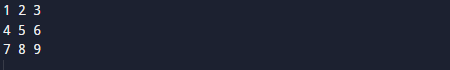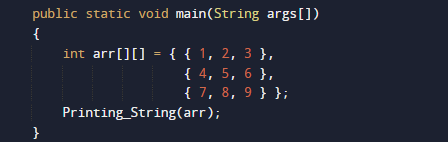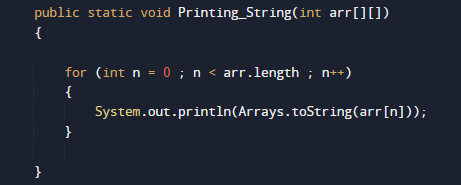# Java Program to Print 2D array

In this tutorial you will learn about the Java Program to Print 2D array and its application with practical example.

In this tutorial, we will learn to create a Java Program to Print 2D array using Java programming.

## Prerequisites

Before starting with this tutorial we assume that you are best aware of the following Java programming topics:

• Java Operators.
• Basic Input and Output function in Java.
• Class and Object in Java.
• Basic Java programming.
• If-else statements in Java.
• For loop in Java.

## What is 2D Array[]?

Multidimensional Arrays Or 2D array defined as in simple words as “array of arrays”.

For example:
The array int[][] a = new int can store a total of (5*5) = 25 elements.

## Java Program to Print 2D array

In this program we would simply print a “2d” array .first of we would declare variables and called a function to display all the value of array[].let have a look at the code

## OutputIn the above program, we have first declared and initialized a set variables required in the program.

• arr[] = it will hold array values.
• n= for iteration

After declaring variables we pass the array to function Printing_String().where we simply print  the array value using for loop.In this tutorial we have learn about the Java Program to Print 2D array and its application with practical example. I hope you will like this tutorial.